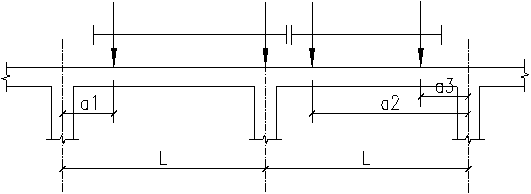### 吊车荷载工程名称:

 吊车数: 2 1 吊车工作级别: A1-A5 A6-A8
 L = m 柱距 [第一台吊车信息] Q = t 吊车起重量，为主钩、副钩总起重量 K = m 吊车轮距 W = m 吊车最大宽度 G = t 吊车总重 g = t 小车重量 Pmax = kN 最大轮压 Pmin = kN 最小轮压

 [第二台吊车信息] Q = t 吊车起重量，为主钩、副钩总起重量 K = m 吊车轮距 W = m 吊车最大宽度 G = t 吊车总重 g = t 小车重量 Pmax = kN 最大轮压 Pmin = kN 最小轮压登录后方可计算,点击登录